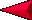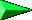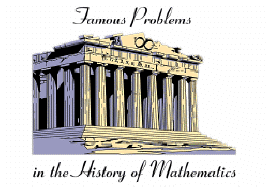Applied Science - Science and Math (6A) Post LabOBJECTIVES: Investigating the early history of mathematics. Exploring how math and science are related. VOCABULARY: algebra arithmetic calculus geometry mathematics trigonometry MATERIALS: worksheet Internet Student use the Internet to find out about fields of mathematics.BACKGROUND: The history of science and math are interrelated.  The people who shaped science are also important in  mathematics.  As in science, the early history of mathematics is sketchy.  We know that the lunar and solar cycles were counted by the Babylonians and Egyptians in an organized fashion.  Early Indian mathematicians are credited with many astronomical observations as well as the beginning of algebra.  The use of decimals and numbers with 9 figures and a zero are also attributed to the Indians.  Their work spread to the Arabic nations, where the term Arabic numbers (compared to Roman numerals) first emerged.  The Chinese are credited with the inventions of spills and abacus, which are both counting machines.  Multiplications tables were used at least from the 6th century BC.  Chinese mathematics were used for the solutions of practical problems in engineering and business.  Although the Chinese had advanced algebra, western scholars seemingly were ignorant of much of this work. Starting in the 6th century BC, Greek mathematicians documented many discoveries in geometry.  The Greeks' conception of numbers as the elements of all things and of the heavens, made mathematical relationships a respected field of study. The philosophy of Pythagoras, Plato and Aristotle reflected the almost "god-like" respect the Greeks gave to the interrelationship of numbers with the universe. Mathematics is a tool of science, but understanding why mathematical formulas work is a science all by itself.  This activity should be used as  a homework assignment or library search.  A good dictionary is also helpful. PROCEDURE:  The internet has many great sites on mathematics.  In order to complete the worksheet have students do a search on different mathematics sites.  Use the key mathematical term to start the search.    ANSWERS: Algebra deals with general statements of relations, utilizing letters and other symbols to represent different values.  Used in science to develop formulas to find an unknown.  Geometry deals with deduction of properties, measurements, and relationships of points, lines, angles, and figures in space.  It includes many different disciplines that try to describe the world.  Used in science to observe and describe. Euclidean geometry deals with a flat world that states that parallel lines are always parallel and never intersect.  Euclidean geometry is our way of measuring on Earth.  Used in science to observe and describe things on Earth. Riemannian Geometry deals with a spherical world that says parallel lines will intersect.  This takes into consideration,  the Universe may be a closed surface.  Used in science to observe and describe throughout the Universe. Trigonometry deals with the relationship between angles and sides.  Used in science to help determine where the location of certain events are, like earthquakes, planets, stars, and many other things that we cannot directly measure. Calculus refers to a large branch in mathematics that can solve many ways to measure (like volume or area) using complicated surfaces.  It is used in most branches of science to derive answers that cannot be measured directly.[Dictionary]   [Back to Applied Science Grid]  [Back to Science & Math (6)]